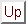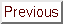Next: Numerical Results of CONDOR. Up: The CONDOR unconstrained algorithm. Previous: Note about the validity   Contents

# The parallel extension of CONDOR

We will use a client-server approach. The main node, the server will have two concurrent process:
• The main process on the main computer is the classical non-parallelized version of the algorithm, described at the beginning of Chapter 6. There is an exchange of information with the second/parallel process on steps 4 and 16 of the original algorithm.
• The goal of the second/parallel process on the main computer is to increase the quality of the model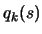by using client computers to sampleat specific interpolation sites.
The client nodes are performing the following:
1. Wait to receive from the second/parallel process on the server a sampling site (a point).
2. Evaluate the objective function at this site and return immediately the result to the server.
3. Go to step 1.
Several strategies have been tried to select good sampling sites. We describe here the most promising one. The second/parallel task is the following:
A.
Make a local copyof(and of the associated Lagrange Polynomials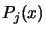)
B.
Make a local copy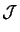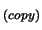of the dataset.
C.
Find the indexof the point insidethe further away from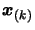.
D.
Replaceby a better point which will increase the quality of the approximation of. The computation of this point is done using Equation 3.38:is replaced inby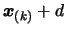whereis the solution of the following problem: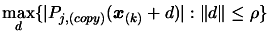(6.7)

E.
Ask for an evaluation the objective function at pointusing a free client computer to performs the evaluation. If there is still a client doing nothing, go back to step C.
F.
Wait for a node to complete its evaluation of the objective function.
G.
Updateusing this new evaluation. Removefrom. go to step C.
In the parallel/second process we are always working on a copy of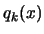,,to avoid any side effect with the main process which is guiding the search. The communication and exchange of information between these two processes are done only at steps 4 and 16 of the main algorithm described in the previous section. Each time the main loop (main process) checks the results of the parallel computations the following is done:
i.
Wait for the parallel/second task to enter the step F described above and block the parallel task inside this step F for the time needed to perform the points ii and iii below.
ii.
Update ofusing all the points calculated in parallel, discarding the points that are too far away from(at a distance greater than). This update is performed using technique described in Section 3.4.3 and Section 3.4.4. We will possibly have to update the index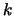of the best point in the datasetand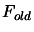.
iii.
Perform operations described in point A & B of the parallel/second task algorithm above:  Copyfrom. Copyfrom.Next: Numerical Results of CONDOR. Up: The CONDOR unconstrained algorithm. Previous: Note about the validity   Contents
Frank Vanden Berghen 2004-04-19